Courses

# Test: Inequalities- 2

## 20 Questions MCQ Test Quantitative Aptitude for SSC CGL | Test: Inequalities- 2

Description
This mock test of Test: Inequalities- 2 for GMAT helps you for every GMAT entrance exam. This contains 20 Multiple Choice Questions for GMAT Test: Inequalities- 2 (mcq) to study with solutions a complete question bank. The solved questions answers in this Test: Inequalities- 2 quiz give you a good mix of easy questions and tough questions. GMAT students definitely take this Test: Inequalities- 2 exercise for a better result in the exam. You can find other Test: Inequalities- 2 extra questions, long questions & short questions for GMAT on EduRev as well by searching above.
QUESTION: 1

### If  4x+2<x2+3x−18<0, where x is an integer, what is the value of x ?

Solution:

Given Info:

• 4x+2<x2+3x−18<0  , where x is an integer.
• We can write the above inequality as two inequalities:
• One is the quadratic inequality given as → x2+3x−18<0 (1st Inequality)
• And the other is also a quadratic inequality given as → 4x+2<x2+3x−18  (2nd Inequality)

To Find:

• Value of x

Approach:

• For finding the possible values of x, we will need to find the range of values of x using the given inequalities.
• We will solve the 1st inequality by using the wavy line method.
• We will solve the 2nd inequality by using the wavy line method.
• From both the inequalities, we will find values of x which simultaneously satisfy both inequalities.

Working out:

1st Inequality

• x2+3x−18<0

⇒x2+6x−3x−18<0

⇒x(x + 6) - 3(x + 6) < 0

⇒  (x + 6)(x - 3) < 0

• The zeroes of the above inequality are x=-6 & x=3
• Plotting the above inequality on the number line and making wavy line for the quadratic inequality (numbers -6 & 3 will not be included in the range of values of x obtained), we get,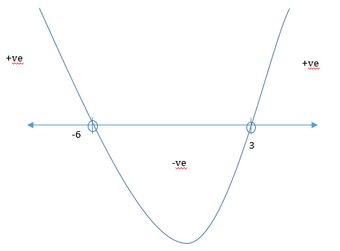• From the above wavy line of the inequality we get x = {-5,-4,-3,-2,-1,0,1,2}, since x is an integer.

2nd Inequality

• x2+3x−18>4x+2

⇒x2+3x−18−4x−2>4x+2−4x−2

⇒x2−x−20>0

⇒x2−5x+4x−20>0

⇒(x−5)(x+4)>0

• The zeroes of the above inequality are x = 5 & x = -4
• Plotting the above inequality on the number line and making wavy line for the quadratic inequality (numbers -4 & 5 will not be included in the range of values of x obtained), we get,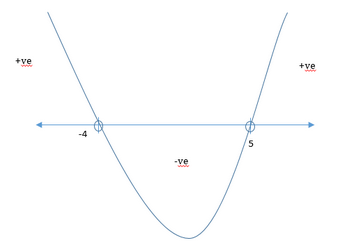• From the above wavy line of the inequality we get x <-4 & x>5, where x is an integer.
• Now from 1st inequality we have, x = {-5,-4,-3,-2,-1,0,1,2} and from 2nd inequality we have x<-4 & x>5.
• Combining the results of both inequalities as shown in the figure below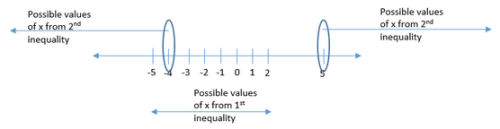• From the above figure we can see that only x=-5 is satisfying both the inequalities.
• So x= -5

QUESTION: 2

### Is the distance between Snape’s home and college greater than 20 kilometers? (1) If Snape drives at a speed of 25 kilometers per hour, he takes less than 1 hour to reach his college from his home. (2) If Snape drives at a speed of 12 kilometers per hour, he takes more than 105 minutes to reach his college from his home

Solution:

Steps 1 & 2: Understand Question and Draw Inferences

Let the distance between Snape’s home and college be d.

We need to find , if d > 20?

Step 3: Analyze Statement 1 independently

(1) If Snape drives at a speed of 25 kilometers per hour, he takes less than 1 hour to reach his college from his home.

d=s∗t

t=d/s  Now we know that the time taken by Snape to reach his college from his home is less than 1 hour. So, t < 1.

As t=d/s , we can write d/s<1

d/25<1

d<25

Insufficient to tell us if d > 20 or not.

Step 4: Analyze Statement 2 independently

(2) If Snape drives at a speed of 12 kilometers per hour, he takes more than 105 minutes to reach his college from his home

d=s∗t

The time taken, t is greater than 105 minutes, which can be written as ((1+45) /60)  hours

So, t > ((1+45) /60)

t >((1+3)/4)

t > 7/4

t=d/s, we have d/12>7/4

d > 21

Sufficient to tell us that d > 20.

Step 5: Analyze Both Statements Together (if needed)

This step is not needed as we have a unique answer from step -4

QUESTION: 3

### For how many values of integer n is 0.01 < 3-n+1 < 0.1?

Solution:

Given:

• Integer n

To find: Number of integral solutions for the inequality 0.01 < 3-n+1 < 0.1

Approach:

• We will first simplify the complicated-looking inequality 0.01 < 3-n+1 < 0.1
• After simplification, we will look at the integer values of n, that are possible.

Working Out:

• Simplifying the given inequality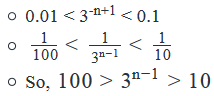• The possible values (integer powers of 3) that lies between 10 and 100 is (33 = 27 & 34 = 81)

Equating 3n-1 to its possible values (33 and 34)

• 3n-1 = 33 ; n -1 = 3 or n = 4
• 3n-1 = 34 ; n -1 = 4 or n = 5
• So, the possible values of n: {4, 5}
• Thus, 2 values of n are possible.

Looking at the answer choices, we see that the correct answer is Option C

QUESTION: 4

Is x + y > 0?

(1) xy > 0

(2) x3y2>0

Solution:

Steps 1 & 2: Understand Question and Draw Inferences

To Find: Is, x+ y > 0?

Step 3: Analyze Statement 1 independently

(1)  xy > 0

• Tells us that x and y have the same sign.
• If x, y > 0, x+ y > 0
• If x, y < 0, x+ y < 0

Step 4: Analyze Statement 2 independently

(2) x3y2>0

• As y2≥0  for all possible values of y, for x3y2>0, x3>0 . So, x > 0
• Also, since the product of x3 and y2 is strictly greater than 0, we can be sure that y is non-zero.
• However, we do not know if y is positive or negative.
• Therefore, we cannot tell is x + y will be positive or negative

Step 5: Analyze Both Statements Together (if needed)

1. From Statement 1:xy > 0
2. From Statement 2:x > 0

Combining both the statements, we have x, y > 0. So, x + y > 0.

QUESTION: 5

Two shopkeepers, Bruce and Wayne, sold the same toy at different prices. If the ratio of the prices at which Bruce and Wayne purchased the toy was 3:4 respectively, who made a greater profit on selling the toy?

(1) The profit percentage of Bruce was higher than that of Wayne.

(2) The ratio of the prices at which Bruce and Wayne sold the toy was 4:3 respectively.

Solution:

Steps 1 & 2: Understand Question and Draw Inferences

• Let the prices at which Bruce and Wayne purchased the toy be 3x and 4x respectively.
• Since 3x and 4x are prices, they must be positive.
• This means, x and y must be positive.
• Let the profit made by Bruce and Wayne be P1 and P2  respectively.

To Find: Which is greater out of Pand P2

Step 3: Analyze Statement 1 independently

(1) The profit percentage of Bruce was higher than that of Wayne.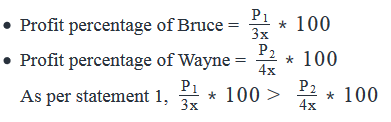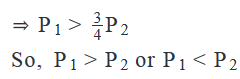(For example, if P1 = 5 and P2 = 4, then P1 > P2 but if P1 = 3.5 and P2 = 4, then P1 < P2. Both these sets of values for P1 and P2 satisfy Statement 1)

Step 4: Analyze Statement 2 independently

(2) The ratio of the prices at which Bruce and Wayne sold the toy was 4:3 respectively.

• Let the prices at which Bruce and Wayne sold the toys be 4y and 3y respectively.
• Profit made by Bruce =P1= 4y – 3x
• Profit made by Wayne =P= 3y – 4x

Assuming, P1 > P2, we have

• 4y – 3x > 3y – 4x
• x + y > 0, which is always true (since x and y are positive).
• This means, our assumption was correct – P1 is indeed greater than P2

Alternate Method

As we can see the selling price of Bruce > selling price of Wayne (4y > 3y) and the cost price of Bruce < cost price of Wayne (3x < 4x).

Thus P1 > P2

Step 5: Analyze Both Statements Together (if needed)

As we have a unique answer from step-4, this step is not required.

QUESTION: 6

How many integers satisfy the inequality x-8 >0, where |x| ≤ 5?

Solution:

Given

• |x| ≤ 5

To Find: Number of integers that satisfy x2 - 8 > 0 ?

Approach

1. For finding the number of integers that satisfy x2 - 8 > 0, we need to first find the range of values of x.
1. Also, for the expression x2 - 8 > 0 we will convert the expression into the form of (x+a)(x-a) > 0 and then use the wavy line method to find out the range of values of x.
2. Also, as we are given |x| ≤ 5, we will find the range of values of x for this inequality.
3. We will then see the overlap region of both the ranges of x to determine the number of integral values of x that satisfy x2 - 8 > 0 .

Working Out

1. |x| ≤ 5

1. The inequality can be interpreted as the distance of x from 0 is less than 5 units, i.e. x is within 5 units from 0. So, we can write,
2. -5 ≤ x ≤ 5

2. x2 - 8 > 0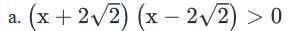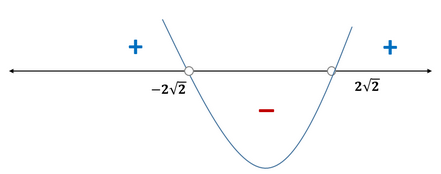3. Hence, the expression is positive for the region x > 2√2 and x < -2√2

1. As √2 ~1.4, 2√2 ~ 2.8
2. So, we can write the expression as x > 2.8 and x < -2.8

4. The integers that satisfy the above and lie between -5 and 5 are {-5, -4, -3, 3, 4, 5}, i.e. a total of 6 integers.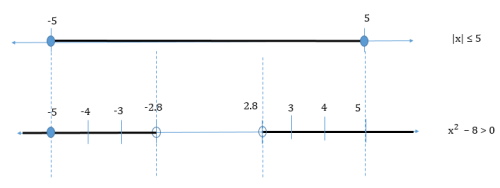QUESTION: 7

If A and B are non-zero numbers such that AB >0, is |A - B| > |A| - |B|?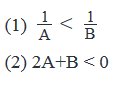Solution:

Steps 1 & 2: Understand Question and Draw Inferences

• Given: AB > -0.
• Either A and B are both positive
• Or A and B are both negative
• To find:  Is |A - B| > |A| - |B|?

Step 3: Analyze Statement 1 independently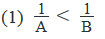• Since AB is positive, multiplying both sides of the inequality with AB will not change the sign of inequality. We get: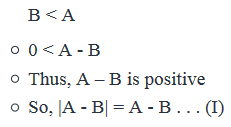• In order to answer the question, we also need to simplify the expression for |A| - |B|
• If A is
• non-negative, then |A| = A
• negative, then |A| = -A
• Similarly for B
• Since we only know that A and B are either both positive or both negative, we’ll have to consider both these cases
• Case 1: A and B are both positive
• This means, |A| = A and |B| = B
• So, |A| - |B| = A - B .. . (II)
• From Equations (I) and (II), we see that
• |A - B| = |A| - |B|
• So, the answer to the question is NO in this case
• Case 2: A and B are both negative
• This means, |A| = -A and |B| = -B
• So, |A| - |B| = -A - (-B) = B - A .. . (III)
• Since A – B is positive, B – A is negative
• Thus, |A| - |B| is negative
• Since a positive number is always greater than a negative number, the answer to the question is: YES

Thus, from the information given in Statement 1, the answer could be YES or NO.

Since we have not been able to determine a unique answer, Statement 1 is not sufficient.

Step 4: Analyze Statement 2 independently

(2) 2A + B < 0

• Remember that in Steps 1 and 2, we’ve inferred that A and B are either both positive or both negative
• If A and B are both positive, then the above inequality is not satisfied (The sum of 2 positive numbers cannot be less than zero)
• Therefore, A and B are both negative
• This means, |A| = -A and |B| = -B
• So, |A| - |B| = -A -(-B) = B - A . .. (I)
• However, we do not know which out of A and B is the bigger number. So, we’ll have to consider both the cases:
• Case 1: A > B
• This means, A – B > 0
• Thus, A – B is positive
• So, |A - B| = A - B . . .  (II)
• In this case, B – A is negative. So, from (I), |A| - |B| is negative
• Since a positive number is always greater than a negative number, the answer to the question is: YES
• Case 2: A≤B
• This means, A−B≤0
• Thus, A –B is not positive
• So, |A−B|=−(A−B)=B−A...(III)
•
• From (I) and (III), |A - B| = |A| - |B|
• Thus, the answer to the question in this case is: NO
• Thus, from the information given in Statement 2, the answer could be YES or NO.
• Since we have not been able to determine a unique answer, Statement 2 is not sufficient.

.

Step 5: Analyze Both Statements Together (if needed)

• From Statement 1: B < A
• If A and B are both positive, answer  = NO
• If A and B are both negative, answer = YES
• From Statement 2: A and B are both negative
• If B < A, answer = YES
• If B ≥ A, answer = NO
• Combining both statements,
• A and B are both negative
• B < A

Since we could arrive at a unique answer, the two statements together are sufficient.

QUESTION: 8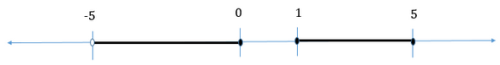Which of the following inequalities have their solutions represented in the shaded part of the number line above?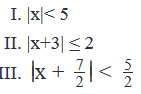Solution:

Given

• A number line graph

To Find: The options that have their solution range represented in the number line graph

Approach

1. We will draw the number line graphs for each of the options and then evaluate if the number line graph covers the solution set of the option

Working Out

1. |x| < 5
1. This inequality refers to all the values of x that are at a distance of less than 5 units from 0 on the number line.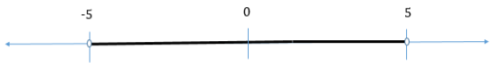The graph in the question statement does not capture the complete region of |x| < 5.

2. |x + 3 | ≤ 2

1. This inequality refers to all the values of x that are at a distance of  less than equal to 2 units from -3 on the number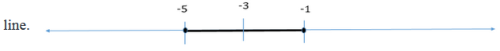The graph in the question statement does not capture the complete region of |x + 3 | ≤ 2

3.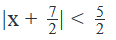• The inequality refers to all the values of x that are at a distance of less than 2.5 units from -3.5 on the number line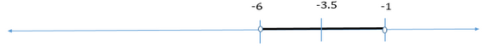The graph in the question statement does not capture the complete region ofAs the number line graph in the question statement does not capture the complete solution region of any of the expressions in the options, answer is option E

QUESTION: 9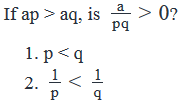Solution:

Steps 1 & 2: Understand Question and Draw Inferences

Given:

• ap > aq
• Case A: a is positive
• Dividing both sides by a will not change the sign of inequality and we’ll get:
• p > q
• Case B: a is negative
• Dividing both sides by a will change the sign of inequality and we’ll get:
• p < q

To find:  Is a/pq>0 ?

• The answer is YES if
• Either a, p and q are all positive
• Or if 2 terms out of a, p and q are negative and the 3rd term is positive

Step 3: Analyze Statement 1 independently

p < q

• This means, Case B from Steps 1 and 2 is applicable. So, we now know that:
• a is negative
• Therefore, a is negative for sure.
• So, the answer will be YES only if one out of p and q is negative and the other is positive
• But we don’t know about the positive/negative nature of p and q

So, Statement 1 alone is not sufficient.

Step 4: Analyze Statement 2 independently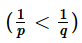• To simplify this inequality, we’ll need to multiply both sides of the inequality with pq
• However, we don’t know the positive/negative nature of pq
• If pq is positive, the sign of the above inequality will not change during simplification
• If pq is negative, the sign of the inequality will reverse during simplification
• Since we don’t know if pq is positive or negative, let’s consider both cases

• Case 1: pq is positive
• This happens if:
• Either p and q are both positive
• Or p and q are both negative
• When both sides of the inequality are multiplied by pq, we get:
• q < p
• In other words, p > q
• This means, Case A from Steps 1 and 2 is applicable. So, we can write:
• a is positive
• Since, a, p and q are all positive, the answer to the question is: YES (as deduced in the ‘To Find’ analysis in Steps 1 and 2)

• Case 2: pq is negative
• This happens if only one out of p and q is positive
• When both sides of the inequality are multiplied by pq, the sign of inequality reverses and we get:
• q > p
• In other words, p < q
• This means, Case B from Steps 1 and 2 is applicable. So, we can write:
• a is negative.
• Thus in this case, 2 terms out of a, p and q are negative and the third one is positive. So, the answer to the question in this case is: YES.

Since we get the same answer – YES – in each of the two possible cases, Statement 2 is sufficient to arrive at a unique answer.

Step 5: Analyze Both Statements Together (if needed)

Since we’ve already arrived at a unique answer in Step 4, this step is not required

QUESTION: 10

If y > x, is y > 64

(1) x  has 7 factors(all even but one) and is divisible by only one prime number.

(2) |y-64| > |x-64|

Solution:

Steps 1 & 2: Understand Question and Draw Inferences

• y > x

To Find: Is y > 64?

Step 3: Analyze Statement 1 independently

(1) x has 7 factors out of which 6 are even factors and one of the odd factors is 1.x is divisible by only one prime number.

• This means, x is of the form:x = p6, where p is a prime number.
• Minimum value of x will be when p will have its minimum value of 2.
• So, possible value of x = 26 = 64
• Since y > x, y > 64

Step 4: Analyze Statement 2 independently

(2) |y-64| > |x-64|

• The above inequality can be interpreted as the distance of  y from 64 on the number line is greater than the distance of x from 64 on the number line.
• So, either y > x > 64 or x < 64 < y
• Please note that there can also be a case where y < x < 64 or y < 64 < x. However, we are given that y > x, so, this case is ruled out.
• Evaluating the possible cases:
• If y > x > 64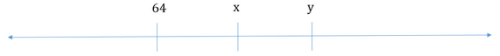In this case y > 64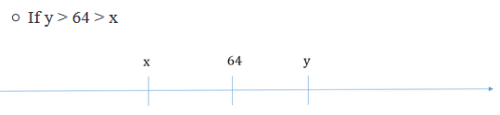• In this case y > 64

Thus, for all the possible cases, y > 64. Sufficient to answer.

Step 5: Analyze Both Statements Together (if needed)

As we have a unique answer from steps 3 and 4, this step is not required.

QUESTION: 11

A group of 10 athletes was competing in a marathon race. If the range of the distance run by the athletes was 10 kilometers, did the total distance run by the athletes exceed 200 kilometers?

(1) The distance covered by one of the athletes was more than 30 kilometers.

(2) The total distance covered by 5 athletes was 125 kilometers.

Solution:

Steps 1 & 2: Understand Question and Draw Inferences

• Let the distances run by athletes be { d1, d2, d3…d10} in ascending order
• d10 – d1 = 10

To Find: If d1 + d2 +  d3…+ d10 > 200?

Step 3: Analyze Statement 1 independently

(1) The distance covered by one of the athletes was more than 30 kilometers.

• Tells us that either of { d1, d2, d3…d10} = 30
• Minimum total distance run by athletes will be if d10 = 30 and d2 = d3…= d10 = 20 (As the range is 10, the minimum distance run by the athletes is 30 – 10 = 20)
• In this case the total distance run will be = 30 + 20 * 9 = 210, which is greater than 200
• So, if even in the case of minimum total distance, the value is greater than 200, we can be sure that for all the other cases, d1 + d2 + d3…+ d10 > 200.
• So, the answer to the question is a definite Yes

Step 4: Analyze Statement 2 independently

(2) The total distance covered by 5 athletes was 125 kilometers.

• As statement-2 tells us the distance run by 5 athletes, for finding the total distance run by 10 athletes, we need to find the range of the possible distance run by the other 5 athletes.
• If 125 kilometers + minimum distance run by other 5 athletes > 200 kilometers, then for all the other cases, the total distance run by the athletes will be greater than 200 kilometers. So, the answer to the question would be YES
• If 125 kilometers + maximum distance run by the other 5 athletes ≤ 200 kilometers, then for all the other cases, the total distance run by the 10 athletes will be less than 200 kilometers. So, the answer to the question would be NO.
• For all the other cases, we would need to know the exact distance run by the other 5 athletes.
• Minimum possible distance run by the 10 athletes:
• As we are given the range of the distance run by athletes to be 10 kilometers, the distance run by the other 5 athletes will be minimum when the distance run by each of the other 5 athletes is 10 less than the maximum possible distance run by any athlete. The lower the maximum distance run by an athlete, the lower the minimum distance run by the other 5 athletes.
• As we are given that the first 5 athletes ran a total distance of 125 kilometers, the lowest maximum possible distance run here would be 25 kilometers by each of the athlete.
• If each of the 5 athletes ran 25 kilometers, the other 5 should run 25 – 10 = 15 kilometers each.
• In this case, the total distance run by the 10 athletes = 125 +75 = 200.
• Hence the total distance run by the athletes was not greater than 200 kilometers.
• Maximum possible distance run by the 10 athletes:
• Using the same logic as above, the maximum distance run by each of the other 5 athletes = 25 + 10 = 35 kilometers
• So, the total distance run by the 10 athletes = 125 + 35*5 = 300 kilometers
• So, 200 ≤ Total distance run by 10 athletes ≤ 300
• If the total distance run by the athletes is 200 kilometers, then the answer to the question is NO
• For all the other cases, where the total distance run by the athletes is greater than 200 kilometers, the answer to the question is YES.

Step 5: Analyze Both Statements Together (if needed)

As we have a unique answer from step-3, this step is not required.

QUESTION: 12

Is x < y?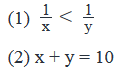Solution:

Steps 1 & 2: Understand Question and Draw Inferences

We need to find if x < y

Step 3: Analyze Statement 1 independently

Statement 1 says that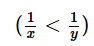If 1/x and 1/y  have the same sign, then the direction of inequality is reversed for their reciprocals, that is, x > y. And so, the answer to the asked question is NO.

If 1/x  and  1/y have the opposite sign, then x < y. The answer is YES.

So, not sufficient to determine a unique answer to the posed question.

Step 4: Analyze Statement 2 independently

Statement 2 says that x + y = 10

This can happen for x = 2 and y = 8 (Here, x < y)

Or for x = 11 and y = -1 (Here, x > y)

So, not sufficient to determine if x < y.

Step 5: Analyze Both Statements Together (if needed)

From St. 1,

The answer to the question depends on whether x and y have the same sign or opposite sign

From the analysis done in St. 2:

x and y can either have the same sign or opposite signs.

So, still not sufficient.

QUESTION: 13

If (x−2)2>1,what is the range of values for x?

Solution:

Given Info:

• (X - 2)2 > 1
• The given expression is a quadratic inequality in x as x is raised to the power of 2.

To Find:

• Range of values of x. We need to calculate the range of those values of x, for which the above inequality will hold true.

Approach:

• For finding the range of values of x, we will solve the above inequality by using the wavy line method.
• After solving the inequality for various values of x, we will get the range of values for which the given inequality is satisfied.

Working out:

• (X - 2)2 > 1
• Subtracting 1 from both sides of the inequality (Subtracting any number in the inequality will not result in any change in sign of the inequality)

⇒  (X - 2)2 - 1> 0

• Expanding (X - 2)2, we get
⇒(x2−4x+4)−1>0
• ⇒x2−4x+3>0⇒x2−3x−x+3>0
• ⇒x(x−3)−1(x−3)>0
• ⇒(x−3)(x−1)>0
• The zeroes of the above inequality are x=3 & x=1
• Plotting the above inequality on the number line and making wavy line for the quadratic inequality (numbers 1 & 3 will not be included in the range of values of x obtained), we get,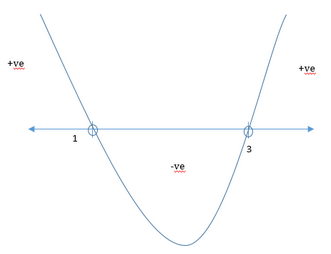• From the above wavy line of the inequality, we can see that x is positive when x>3 or x<1.

• So the range of values of x are x>3 or x<1.

Hence answer option D is correct.

QUESTION: 14

If (|x| -1)2 > 4, which of the following may be true?

I. |x| < 1

II. |x +1| < 1

III. |x -2|2 ≥ 4

Solution:

Given:

• (|x| -1)2 > 4

To Find: The options that may be true for the given inequality

Approach

1. We will simplify the inequality (|x|−1)2−22>0  and then use the formula of a2 - b2 >0 to simplify it further and find the range of x.  Let’s call this range as S.
2. Then, we’ll solve the inequalities in the options and find their ranges.
1. The inequality in the option may be true if its range has any overlap with S.

Working Out

1. Solving the inequality (|x| -1)2 > 4

​​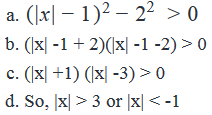2. Solving the reduced inequality

1. |x| > 3. So, x > 3 or x < -3
1. The inequality above refers to all the values of x that are at a distance of greater than 3 units from 0 on the number line.
2. |x| < -1. This is not possible as |x| ≥ 0

So, the inequality is true for x > 3 and x < -3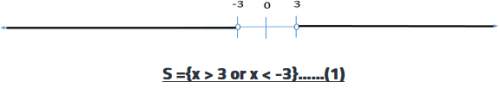I. |x| < 1

1. The inequality refers to all the values of x that are at a distance of less than 1 unit from 0 on the number line.
2. -1 < x < 1
3. The range -1 < x < 1 has no overlap with range S.
4. Hence Option I is never true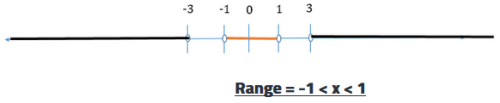II. |x +1| < 1.

1. The inequality refers to all the possible values of x that are at a distance of less than 1 unit from -1 on the number line. So, -2 < x < 0
2. You can also solve it algebraically as below:
3. Two cases are possible:
4. If x+1 ≥ 0, i.e. x ≥ -1
1. x +1 < 1, i.e. x < 0. So, we have -1 ≤ x < 0
5. If x +1 < 0, i.e. x < -1
1. x +1 > -1, i.e. x > -2. So, we have -2 < x < -1
6. Combining the two ranges, we have -2 < x < 0.
7. The range -2 < x < 0 does not have any overlap with S. Hence, option II is never true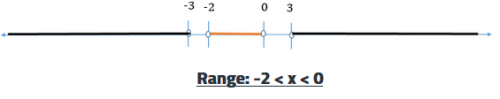III. |x -2|2 ≥ 4.

1. Solving the inequality to |x−2|2−22>0
2. (|x-2| -2) (|x-2| +2) ≥ 0
3. Two cases are possible: |x-2| ≥ 2 or |x-2| ≤ -2
4. |x-2| ≥ 2.
1. The inequality refers to all the values of x that are at a distance of greater than 2 units from 2 on the number line
2. So, x ≥ 4 or x ≤ 0
3. It can also be solved algebraically as below
4. If x -2 ≥ 0, i.e. x ≥ 2
1. x -2 ≥ 2, i.e. x ≥ 4
Please note that x ≥ 4 has some overlap with the range S, so option III may be true for some values of x. Hence, there is no need to analyse the further cases. However we are analysing the other cases for the sake of completing the analysis.
5. If x – 2 < 0, i.e. x < 2
1. –x + 2 ≥ 2, i.e. x ≤ 0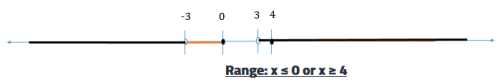5. |x-2| ≤ -2.

1. We can see from the inequality above that the left hand side is always non-negative. So, it can never be less than a negative integer. Hence, this inequality is not true for all any value of x.
2. It can also be solved algebraically as below:
3. If x – 2 ≥ 0, i.e. x ≥ 2
1. x – 2 ≤ -2, i.e. x ≤ 0. Not possible as we have assumed x ≥ 2
4. If x – 2 < 0, i.e. x < 2
1. –x + 2 ≤ -2, i.e. x ≥ 4. Not possible as we have assumed x < 2.

So, the inequality is true for x ≤ 0 and x ≥ 4. This range has some overlap with S.

Hence option III may be true for some values of x.

QUESTION: 15

If x2≤16andx2>4, how many integral values of x are possible?

Solution:

Given

• x2≤16  and x2>4

To Find: Integral values of x that satisfy both the inequalities

Approach

1. We will convert the inequality x2≤16

into (x+a) (x-a) ≤ 0 form and the inequality x2>4

1.  into (x+b)(x-b) > 0 form and then draw the wavy line diagram to find out the integral values fo x that satisfy both the inequality.

Working Out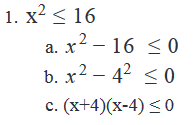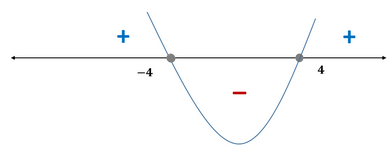d. So, the inequality is true for range -4 ≤ x ≤ 4

e. Hence, the integral values of x in the range = {-4, -3, -2, -1, 0, 1, 2, 3, 4}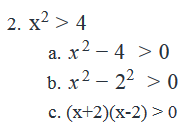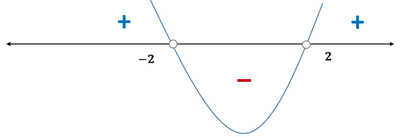d. So, the inequality is true for range when x < -2 or x > 2

e. Hence, the integral values of x in the range = {-∞…….-4, -3, 3, 4, ………+∞}

3. The integral values of x that satisfy both the inequality are = { -4, -3, 3, 4}, i.e. 4 values.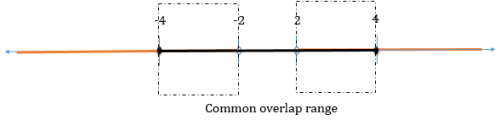QUESTION: 16

If a, b, c and d are positive consecutive multiples, not necessarily in that order, of a positive integer x greater than 1, is a + b + c + d ≥ 50?

(1) c = 15

(2) The difference between d and b is divisible by only four positive integers, one of which is 10.

Solution:

Steps 1 & 2: Understand Question and Draw Inferences

• x is an integer > 1
• a, b , c, d are integers > 0
• Let the least multiple of x in {a, b, c , d} be xy, where y > 0
• We are taking the least multiple to be xy because we do not know which integer out of a, b, c and d is the least in value. Of course, xy will be equal to one integer out of a, b, c or d.
• So, we can express the other 3 multiples of x in terms of y as: x(y+1), x(y+2), x(y +3)

To Find: Is a + b + c + d ≥ 50?

That is, is xy + x(y+1) +x(y+2) +x(y+3) ≥ 50 ?

Is 4x +6xy  ≥ 50 ?

That is, is x(2y + 3) ≥  25?

So, we need to find a unique answer to the question is x(2y + 3) ≥  25?

Step 3: Analyze Statement 1 independently

(1) c = 15

As c is a multiple of x, the value of x can be the factors of 15  greater than 1= {3, 5, or 15}. We need to see, if for a value of x, is x(2y+3) ≥ 25?

• If x = 15
• We need to calculate the minimum possible value of y, keeping in mind the constraint that one of the integers, i.e. c = 15
• Minimum possible value of y = 1. For such a case, we will have xy = c = 15. So, minimum possible value of y = 1.
• Minimum value of x(2y+3) = 75 > 25.
• For all values of y, x(2y+3) ≥ 25.
• Is, a + b +c + d ≥ 50> → Yes
• If x = 5
• Minimum Value of y = 1. In this case we will have x(y+2) = c = 15. So, minimum possible value of y = 1
• Minimum value of x(2y+3) = 25 = 25
• For values of y ≥ 1, x(2y+3) ≥ 25
• Is, a +b+c+d ≥ 50> → Yes
• If x = 3
• If we assume here that the minimum possible of y = 1, the maximum possible number out of (a,b,c,d) will be x *(y+3) = 12. So, y = 1 is not the minimum possible value of y.
• For x(y+3) = 15, we need to have a minimum possible value of y + 3 = 5, i.e. y =2
• So, minimum of x(2y+3) = 21 < 25
• For values of y ≥ 3, x(2y+3) ≥ 25
• Is, a +b+c+d ≥ 50> → Yes/No

As we do not have a unique answer to the question Is, a +b+c+d ≥ 50 , the statement is insufficient to answer.

Step 4: Analyze Statement 2 independently

(2) The difference between d and b is divisible by only four positive integers, one of which is 10.

The possible values of difference between d and b can be ={x, 2x, or 3x}. As we are given that the difference between d and b is divisible by 10, we will try to find , if for all values of x and y, is x(2y+3) ≥ 25?

Also, as 10 has four factors (1,2,5 and 10), a number, which is divisible by 10 will be divisible by all the factors of 10. Since |d-b| is divisible by 10 and has only 4 factors, the only possible value of |d-b| = 10

• Case-I:|d – b| = x, i.e. d and b are consecutive multiples. So, x = 10.
• Minimum value of y = 1
• Minimum value of x(2y+3) = 50 > 25
• For all values of y, x(2y+3) ≥ 25.
• Is, a + b +c + d ≥ 50> → Yes

• Case-II:|d-b| = 2x = 10, i.e. x = 5
• Minimum value of y = 1
• Minimum value of x(2y+3) = 25 = 25
• For values of y ≥ 1, x(2y+3) ≥ 25
• Is, a +b+c+d ≥ 50> → Yes

• Case-III:|d-b| = 3x = 10. Not possible as 10 is not divisible by 3

As we have a unique answer to the question Is, a +b+c+d ≥ 50 , the statement is sufficient to answer.

Step 5: Analyze Both Statements Together (if needed)

As we have a unique answer from step 4, this step is not required

QUESTION: 17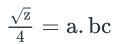The decimal representation of the number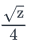is given above, where z is a positive number and a, b and c are single-digit non-negative integers. Is a = 0?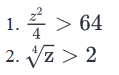Solution:

Steps 1 & 2: Understand Question and Draw Inferences

Given: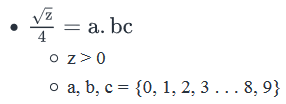To find: Is a = 0?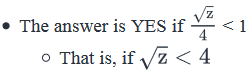• Since √z is positive, the sign of inequality will remain the same upon squaring both sides
• So, the answer is YES if z < 16
• Else, the answer is NO

Step 3: Analyze Statement 1 independently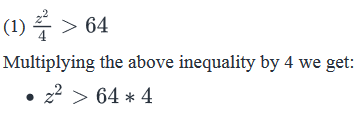Taking square roots we get

• z > 8 * 2 (Ignore negative roots as z is given as positive number)
• z > 16
• So, z is not less than 16
• So, the answer is NO

Statement 1 is sufficient to answer the question

Step 4: Analyze Statement 2 independently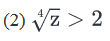Since 4 is positive, squaring both sides twice will not change the sign of inequality: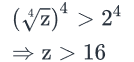• So, z is not less than 16
• So, the answer is NO

Statement 2 is sufficient to answer the question

Step 5: Analyze Both Statements Together (if needed)

Since we’ve already arrived at a unique answer in Steps 3 and 4, this step is not required

QUESTION: 18

If |p-5| =3 and |q-3| = 5, which of the following statements must be true?

Solution:

Given

• |p – 5| = 3
• |q – 3| = 5

To Find: The options that must be true(for all values of p and q)

Approach

1. Since we are given expressions in p and q, we will find the possible values of p and q and then evaluate each of the expression in the options given for their trueness.
1. Since, we need to look for must be true statements, any case that makes the expression in the option false will rule out the option.

Working Out

1. As | p – 5| = 3, value of p will be 3 units from away from 5 on the number line. So, following can be values of p:

1. p = 5 + 3 = 8 or
2. p = 5 – 3 = 2
3. So, p = { 2, 8}……….(1)

2. As | q – 3| = 5, value of q will be 5 units away from 3 on the number line. So, following can be values of q:

1. q = 3 + 5 = 8 or
2. q = 3 – 5 = -2
3. So, q = {-2, 8}………(2)

3. Evaluating Options

1. p + q > 0 → As p + q = 0, when p = 2 and q = -2, this statement is not always true.
2. pq ≥ 0→ Both the possible values of p are positive. For value of q = -2, the value of pq < 0. Hence this statement is not always true
3. |p| = |q|→ This statement is not always true, as |p| can be 2 when |q| = 8 or vice versa.
4. |p| ≥ |q|→ This statement is not always true, as |p| can be 2 when |q| = 8, in which case we will have |p| < |q|
5. -6 ≤ p-q ≤ 10→ We need to find the minimum and maximum value of p – q
1. Minimum( p –q) = Minimum p – Maximum q = 2 – 8 = -6
2. Maximum(p – q) = Maximum p – Minimum q = 8 – (-2) = 10
3. So, value of p –q would always lie between -6 and 10, inclusive. Hence, this statement is always true.

QUESTION: 19

How many integrals value of x satisfy the inequality (1-x2)(4-x2)(9-x2) > 0 ?

Solution:

Given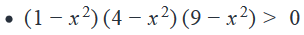To Find: Integral values of x that satisfy the inequality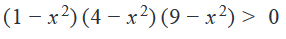Approach

1. We will draw the wavy line diagram to find the range of x. In the range of x we will observe the number of integral values of x that satisfy the inequality.
a. Before we draw the wavy line diagram, we should have the coefficient of x as positive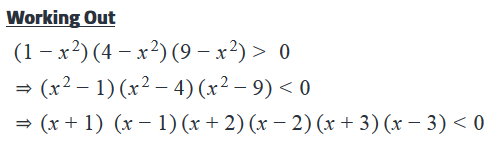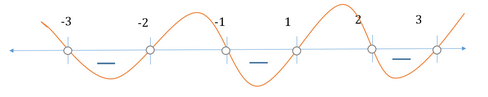As we can see from the wavy line diagram that the inequality is true for the following range:

1. -3 < x < -2→ Integral values of x = {Nil}
2. -1 < x < 1 → Integral values of x = {0}
3. 2 < x < 3 → Integral values of x = {Nil}

Thus, there is only 1 integral value of x that satisfy the inequality i.e. 0.

QUESTION: 20

A bag contains red balls that weigh 100 grams each and green balls that weigh 50 grams each. If the number of green balls is 9 more than the number of red balls, how many balls are there in the bag?

(1) If two red balls are added to the bag, the number of red balls will be half the number of green balls

(2) The total weight of the balls in the bag is between 1.05 kilogram and 1.35 kilogram

Solution:

Steps 1 & 2: Understand Question and Draw Inferences

Let the number of red balls be R and the number of green balls be G.

Note that R and G must be non-negative integers, since these denote the number of balls.

Given: G = R + 9 .  . . (I)

And, weight of each Green ball = 50 grams

Weight of each Red ball = 100 grams

Need to find: G + R

Step 3: Analyze Statement 1 independently

Statement 1 says that if two red balls are added to the bag, the number of red balls will be half the number of green balls

R+2=G/2

2R + 4 = G . . . (II)

Equations (I) and (II) form 2 linear equations with 2 unknowns. Sufficient to find R and G.

Step 4: Analyze Statement 2 independently

The total weight of the balls in the bag is between 1.05 kilogram and 1.35 kilogram​

1.05 kg = 1050 grams

1.350 kg = 1350 grams

Statement 2 says that

1050< 100R + 50G < 1350

105 <10R+5G < 135

21 < 2R + G < 27

Using (I)

21 < 3R + 9 < 27

7 < R + 3 < 9

4 < R < 6

• R = 5 (since R must be an integer)
• G = 14 (from Eq. I)

Sufficient.

Step 5: Analyze Both Statements Together (if needed)

Since we arrive at a unique answer in each of Steps 3 and 4, this step is not required